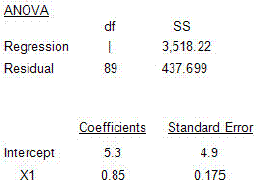### CFA Practice Question

There are 208 practice questions for this study session.

### CFA Practice Question

In comparing the relation of company sales with GDP, your regression software provides the following output:What must be the coefficient of determination of this regression equation:
A. 73.4%
B. 87.6%
C. 89.0%
Explanation: R2 = RSS/TSS = (3,518.22)/(3,518.22 +437.699) = 89%.